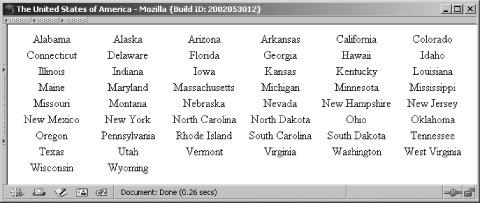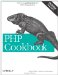# Recipe 4.25. Program: Printing a Horizontally Columned HTML Table

Converting an array into a horizontally columned table places a fixed number of elements in a row. The first set goes in the opening table row, the second set goes in the next row, and so forth. Finally, you reach the final row, where you might need to optionally pad the row with empty table data cells.

The function pc_grid_horizontal( ), shown in Example 4-4, lets you specify an array and number of columns. It assumes your table width is 100%, but you can alter the \$table_width variable to change this.

##### pc_grid_horizontal( )

 `function pc_grid_horizontal(\$array, \$size) { // compute
width %ages \$table_width = 100; \$width = intval(\$table_width / \$size); // define how our
tags appear // sprintf() requires us to use %% to get literal % \$tr = '
%s
and '; \$td = ""; // open table \$grid = "\$tr"; // loop through entries and display in rows of size \$sized // \$i keeps track of when we need a new table tow \$i = 0; foreach (\$array as \$e) { \$grid .= sprintf(\$td, \$e); \$i++; // end of a row // close it up and open a new one if (!(\$i % \$size)) { \$grid .= "\$tr"; } } // pad out remaining cells with blanks while (\$i % \$size) { \$grid .= sprintf(\$td, ' '); \$i++; } // add , if necessary \$end_tr_len = strlen(\$tr) * -1; if (substr(\$grid, \$end_tr_len) != \$tr) { \$grid .= ''; } else { \$grid = substr(\$grid, 0, \$end_tr_len); } // close table \$grid .= ''; return \$grid; }`

The function begins by calculating the width of each <td> as a percentage of the total table size. Depending on the number of columns and the overall size, the sum of the <td> widths might not equal the <table> width, but this shouldn't affect the displayed HTML in a noticeable fashion. Next, define the <td> and <tr> tags, using printf-style formatting notation. To get the literal % needed for the <td> width percentage, use a double %%.

The meat of the function is the foreach loop through the array in which we append each <td> to the \$grid. If you reach the end of a row, which happens when the total number of elements processed is a multiple of the number of elements in a row, you close and then reopen the <tr>.

Once you finish adding all the elements, you need to pad the final row with blank or empty <td> elements. Put a non-breaking space inside the data cell instead of leaving it empty to make the table render properly in the browser. Now, make sure there isn't an extra <tr> at the end of the grid, which occurs when the number of elements is an exact multiple of the width (in other words, if you didn't need to add padding cells). Finally, you can close the table.

For example, let's print the names of the 50 U.S. states in a six-column table:

`// establish connection to database \$dsn = 'mysql://user:password@localhost/table'; \$dbh = DB::connect(\$dsn); if (DB::isError(\$dbh)) { die (\$dbh->getMessage()); } // query the database for the 50 states \$sql = "SELECT state FROM states"; \$sth = \$dbh->query(\$sql); // load data into array from database while (\$row = \$sth->fetchRow(DB_FETCHMODE_ASSOC)) {   \$states[] = \$row['state']; } // generate the HTML table \$grid = pc_grid_horizontal(\$states, 6); // and print it out print \$grid;`

When rendered in a browser, it looks like Figure 4-1.

##### The United States of AmericaBecause 50 doesn't divide evenly by 6, there are four extra padding cells in the last row .PHP Cookbook: Solutions and Examples for PHP Programmers
ISBN: 0596101015
EAN: 2147483647
Year: 2006
Pages: 445

Similar book on Amazon